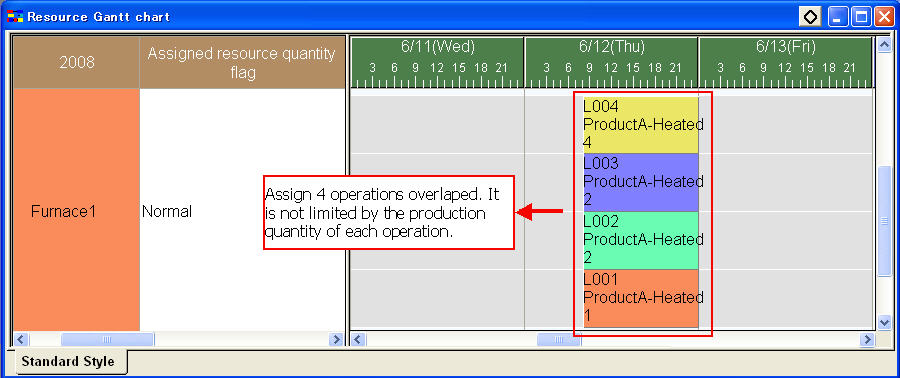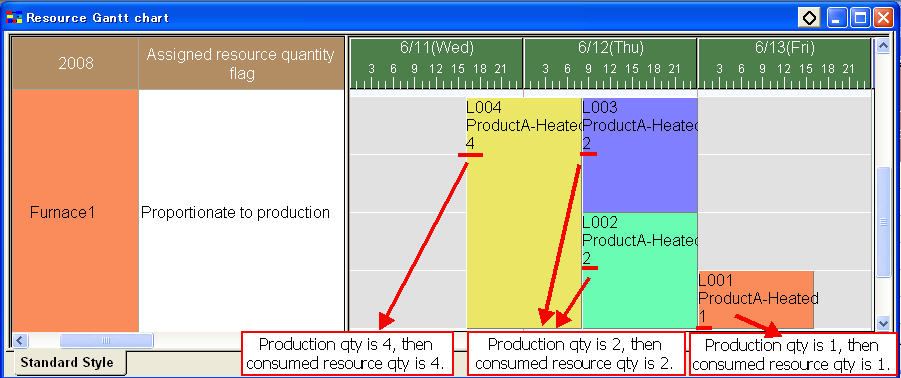## Assigned resource quantity flag

For example, there is limitation of the furnace capacity when dealing several operations at the same time in Furnace data. There are two examples:

1. Put 10 operations into furnace at the same time. Operations' account limitation.(No relationship with production quantity.)
2. Put 10 ProductA into furnace at the same time. Proportionate to production quantity.

In particular, the volume and weight of item will take negative effects to deal with all of them at the same time in the furnace. There is a maximum limitation of the operations' quantity when dealing at the same time.

In case that there is a limitation about the production quantity of operations or the operations quantity. For the production quantity limitation, set "Assigned resource quantity flag"property of resource as "Normal" to deal with it. For the other one, set "Assigned resource quantity flag"property of resource as "Proportionate to production quantity" and then it's OK.

There are two options for "Assigned resource quantity flag"property.

SettingsMeaning
NormalOne operation will consume the required resource quantity which is registered in master.
Proportionate to production quantityCalculate the consumed resource quantity according to the production quantity of operations and the required resource quantity which is registered in master.

Example

There are 4 Furnace1s in calendar table.
The required resource quantity registered in master is 1.

In case that the maximum limitation of Furnace1 at the same time is 4. Set "Assigned resource quantity flag"property of resource as "Normal".
At most 4 operations can be done at the same time in Furnace1, no matter the production quantity of operations.In case that the maximum limitation of Furnace1 at the same time is 4. Set "Assigned resource quantity flag"property of resource as "Proportionate to production quantity".
The production quantity of order L004 is 4. The required resource quantity is 1. Then consumed resource quantity is 4*1=4. The maximum limitation of Furnace1 is 4. Furnace1 cannot do other operations when doing L004.
The production quantity of order L003 and L002 are 2. The required resource quantity is 1. Then consumed resource quantity is (2+2)*1=4. The maximum limitation of Furnace1 is 4. L002 and L003 can be done at the same time in Furnace1.
The production quantity of order L001 is 1. The required resource quantity is 1. Then consumed resource quantity is 1*1=1. The maximum limitation of Furnace1 is 4. There are still 3 unit free resource in Furnace1.* "Assigned resource quantity flag" property is not only use in furnace example but also can be used by other samples.